Next: Classification of Subgroups of Up: A Symmetry Classification of Previous: Introduction

# Symmetries of Columns

We define a column by a real-valued function f on the cylinder. Let. The functionmeasures the height of the column in the direction normal to the cylinder at the point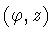.

The group of symmetries of the cylinder iswhereacts onbyMultiplication infollows from the definition of the action. Suppose that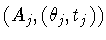is infor j=1,2, where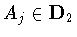,and. Then multiplication is given by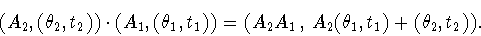(1)

We wish to classify columns by their symmetries. A symmetry of the column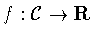issuch thatThe symmetry groupis the collection of all symmetries of f. We classify all subgroups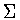which are symmetry subgroups for some column f.

Our classification proceeds as follows. To each subgroup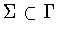, we can associate the normal subgroup(2)

(Soconsists of the pure `translations' in.) Thus it suffices to
(i)
classify the closed subgroupsof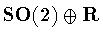,
(ii)
for each subgroupin (i), compute the subgroupsthat satisfy (2.2).
The calculation in (ii) is simplified by observing thatis contained in the normalizer of.

As usual, we identify conjugate subgroups of. In addition, we identify subgroups that are related by axial scalings. More precisely, we define the scaling transformationbyProvided, this is an isomorphism. We say that two subgroups,are related by a scaling iffor some nonzero.Next: Classification of Subgroups of Up: A Symmetry Classification of Previous: Introduction
Marty Golubitsky
2001-01-29